# How to use the DEVSQ Function in Excel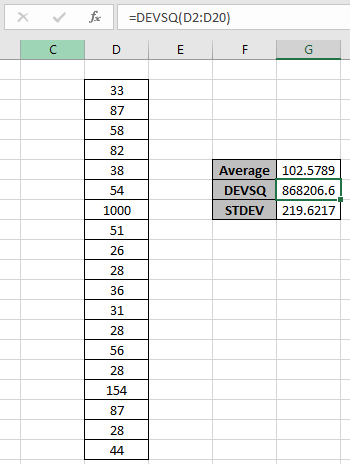In this article, we will learn about how to use the DEVSQ function in Excel that returns the sum of squares of deviations of data points from their sample mean.

What is the DEVSQ function? What is sum of squared deviations?

DEVSQ function is a built-in function to calculate the sum of squared deviations from the mean or average of the range of data values provided. The DEVSQ for the set of values is calculated using the formula shown below.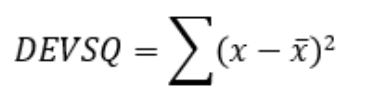Here:

1. x is the number.
2. x s the mean or average of the range.

DEVSQ function in excel returns a number which is the sum of squared deviation from average or mean of the input numbers dataset

Syntax of DEVSQ function:

=DEVSQ (value 1, [value 2], …)

value 1 : First value ( necessary )

value 2 : Second value ( optional ). The values can be given using cell reference.

Example:

All of these might be confusing to understand. So, let's test this formula via running it on the example shown below. Here we have some random data values to test the DEVSQ function in Excel.

Here we have a range of values from E2:E20 cell and we need to get the sum of squared deviation from average or mean for the same range using the below formula

Use the formula:

=DEVSQ(E2:E20)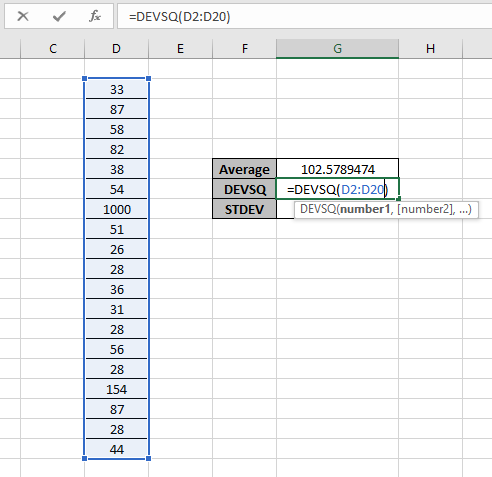See the formula in the cell. Here array value arguments to the function are given using the cell reference method. Using the above formula in the cell to get the DEVSQ of the range values.The sum of squared deviation from the Average or mean for the random data values considering all number values using DEVSQ is 868206.6 and AVERAGE function returns the same result 102.5789 for the same data set values.

Let's see how the funtion returns the result.
First of all take the average of the range in G6 cell. Then take the difference with the mean with each value in range and square it.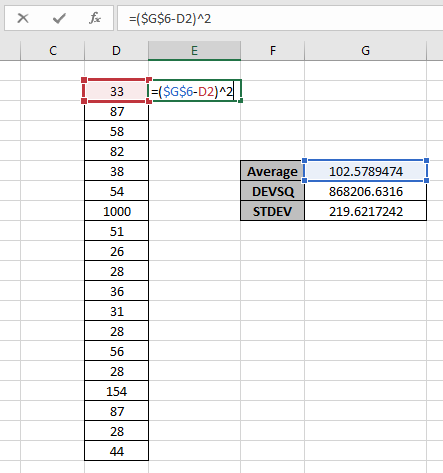Use the formula:

=(G6 - D2) ^ 2

Here G6 is freezed at \$G\$6 cell which is the mean or average of the range. And D2, D3 will be each value squared of the value using ( ^ 2).

Now Take the sum of the values.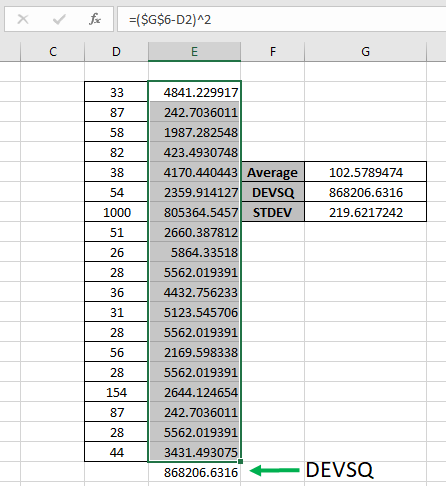Both values return the same result. And this is what the DEVSQ function does.

Here are some observational notes shown below.

Notes :

1. The function takes 255 values as maximum count.
2. The function ignores all values except numbers in number format.
3. The blank cells are ignored but the value 0 is considered and considered as 0.
4. The function considers some data set number as date, time, etc. So Careful using the values with date or time values.
5. If data has some particular criteria, use the AVERAGEIF function & AVERAGEIFS function.

Hope this article about How to use the DEVSQ function in Excel is explanatory. Find more articles on statistical function formulas here. If you liked our blogs, share it with your fristarts on Facebook. And also you can follow us on Twitter and Facebook. We would love to hear from you, do let us know how we can improve, complement or innovate our work and make it better for you. Write to us at info@exceltip.com.

Related Articles

How to use the AVERAGE function in Excel : AVERAGE function is used in Excel for values considering only numbers which are in number format.

How to use the AVERAGEIF function in Excel : This function takes the average of values in range applying any one condition over the corresponding or the same range.

How to use the AVERAGEIFS function in Excel : This function takes the average of values in range applying any multiple conditions over the corresponding or the same range.

How to Use STDEV Function in Excel : Calculate the standard deviation for the data numbers in excel using the STDEV function.

How to use the VAR function in Excel : Calculate the variance for the sample data numbers in excel using the VAR function.

Popular Articles:

50 Excel Shortcuts to Increase Your Productivity | Get faster at your task. These 50 shortcuts will make you work even faster on Excel.

The VLOOKUP Function in Excel | This is one of the most used and popular functions of excel that is used to lookup value from different ranges and sheets.

COUNTIF in Excel 2016 | Count values with conditions using this amazing function. You don't need to filter your data to count specific values. Countif function is essential to prepare your dashboard.

How to Use SUMIF Function in Excel | This is another dashboard essential function. This helps you sum up values on specific conditions.

Terms and Conditions of use

The applications/code on this site are distributed as is and without warranties or liability. In no event shall the owner of the copyrights, or the authors of the applications/code be liable for any loss of profit, any problems or any damage resulting from the use or evaluation of the applications/code.